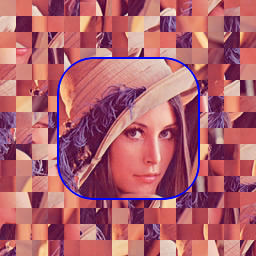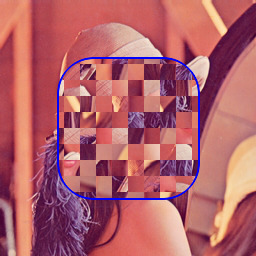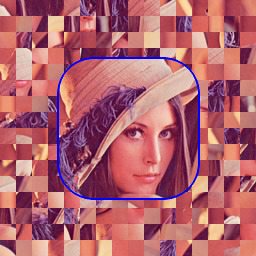## SHUFFLE

 Randomly shuffles positions of square sized tiles in an image.

### EXAMPLES

 Original:Arguments: (default)Original:Arguments: -p 25Original:Arguments: -s 16 -c 128,128 -r 70,70 -R 35,35 -e blueOriginal:Arguments: -s 16 -c 128,128 -r 70,70 -R 35,35 -e blue -nOriginal:Arguments: -s 16 -c 128,128 -r 70,70 -R 35,35Original:Mask:Arguments: -s 16 -e blueOriginal:Arguments: (defaults)What the script does is as follows: Crops the image to a multiple of the dimension Does a dimension-sized tiled crop of image Randomly sorts the tiles Montages all the tiles back to form the output image This is equivalent to the following IM commands wh=`convert -ping "\$infile" -format "%wx%h" info:` ww=`echo "\$wh" | cut -dx -f1` hh=`echo "\$wh" | cut -dx -f2` ww=`convert xc: -format "%[fx:\$dim*floor(\$ww/\$dim)]" info:` hh=`convert xc: -format "%[fx:\$dim*floor(\$hh/\$dim)]" info:` numw=\$((ww/dim)) numh=\$((hh/dim)) num=\$((numw*numh)) convert -quiet "\$infile" -crop \${ww}x\${hh}+0+0 +repage -crop \${dim}x\${dim} \$tmpA1 list="" list=`for ((i=0; i